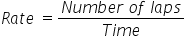Mathematics
Easy

Question

# Meera takes 12 minutes to swim 4 laps. ___________ hours are taken by Meera to swim 45 laps at the same rate.

## 125130135127Hint:

## The correct answer is: 135

### Time taken by Meera to swim 4 laps is 12 minutes.We have to find the rate at which Meera swims. We will find it by taking the ratio of the number of laps and the time required to cover the laps. As the rate is constant, we will use this value to find the time required to cover 45 laps.So the equation becomesSo the rate = 1/3 laps per minute.Let’s find the time taken by Meera to swim 45 laps.We will rearrange this equation for time .For this we will multiply both sides by time and divide by rate.Therefore, time taken my Meera to cover 45 laps is 135 minutes.

We have to be careful about the units. We have to make sure same quantities have same unit

### Related Questions to study#### With Turito Foundation.#### Get an Expert Advice From Turito.